### Home > CCA > Chapter 8 > Lesson 8.2.5 > Problem8-107

8-107.
1. Use factoring and the Zero Product Property to solve each equation. Homework Help ✎

1. (x − 4)(2x + 1) = 0

2. x2 + 5x + 6 = 0

3. x(2x − 5) = 0

4. x2 + 4x = 0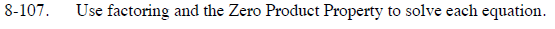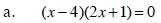What values of x would satisfy this equation? Note that there are two answers.

$\textit{x}=4\text{ or }\textit{x}=-\frac{1}{2}$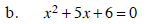Factor and find the values of x that satisfy this equation.

x = −3 or x = −2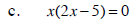Look at the hints for parts (a) and (b) if you need assistance.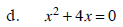Look at the hints for parts (a) and (b) if you need assistance.